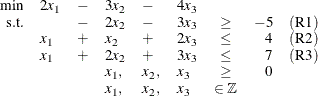The OPTMILP Procedure

Getting Started: OPTMILP Procedure

The following example illustrates the use of the OPTMILP procedure to solve mixed integer linear programs. For more examples, see the section Examples: OPTMILP Procedure. Suppose you want to solve the following problem:The corresponding MPS-format SAS data set follows:

data ex_mip;
input field1 $field2$ field3 $field4 field5$ field6;
datalines;
NAME        .      EX_MIP      .   .        .
ROWS        .        .         .   .        .
N           COST     .         .   .        .
G           R1       .         .   .        .
L           R2       .         .   .        .
L           R3       .         .   .        .
COLUMNS     .        .         .   .        .
.           MARK00 'MARKER'    .   'INTORG' .
.           X1     COST        2   R2       1
.           X1     R3          1   .        .
.           X2     COST       -3   R1      -2
.           X2     R2          1   R3       2
.           X3     COST       -4   R1      -3
.           X3     R2          2   R3       3
.           MARK01 'MARKER'    .   'INTEND' .
RHS         .      .           .   .        .
.           RHS    R1         -5   R2       4
.           RHS    R3          7   .        .
ENDATA      .      .           .   .        .
;


You can also create this SAS data set from an MPS-format flat file (ex_mip.mps) by using the following SAS macro:

   %mps2sasd(mpsfile = "ex_mip.mps", outdata = ex_mip);


This problem can be solved by using the following statement to call the OPTMILP procedure:

proc optmilp data = ex_mip
objsense   = min
primalout  = primal_out
dualout    = dual_out
presolver  = automatic
heuristics = automatic;
run;


The DATA= option names the MPS-format SAS data set that contains the problem data. The OBJSENSE= option specifies whether to maximize or minimize the objective function. The PRIMALOUT= option names the SAS data set to contain the optimal solution or the best feasible solution found by the solver. The DUALOUT= option names the SAS data set to contain the constraint activities. The PRESOLVER= and HEURISTICS= options specify the levels for presolving and applying heuristics, respectively. In this example, each option is set to its default value AUTOMATIC, meaning that the solver automatically determines the appropriate levels for presolve and heuristics.

The optimal integer solution and its corresponding constraint activities, stored in the data sets primal_out and dual_out, respectively, are displayed in Figure 11.1 and Figure 11.2.

Figure 11.1: Optimal Solution

 The OPTMILP Procedure Primal Integer Solution

Obs Objective
Function ID
RHS ID Variable
Name
Variable
Type
Objective
Coefficient
Lower
Bound
Upper
Bound
Variable
Value
1 COST RHS X1 B 2 0 1 0
2 COST RHS X2 B -3 0 1 1
3 COST RHS X3 B -4 0 1 1

Figure 11.2: Constraint Activities

 The OPTMILP Procedure Constraint Information

Obs Objective
Function ID
RHS ID Constraint
Name
Constraint
Type
Constraint
RHS
Constraint
Lower
Bound
Constraint
Upper
Bound
Constraint
Activity
1 COST RHS R1 G -5 . . -5
2 COST RHS R2 L 4 . . 3
3 COST RHS R3 L 7 . . 5

The solution summary stored in the macro variable _OROPTMILP_ can be viewed by issuing the following statement:


%put &_OROPTMILP_;



This produces the output shown in Figure 11.3.

Figure 11.3: Macro Output

 STATUS=OK   ALGORITHM=BAC   SOLUTION_STATUS=OPTIMAL   OBJECTIVE=-7 RELATIVE_GAP=0   ABSOLUTE_GAP=0   PRIMAL_INFEASIBILITY=0 BOUND_INFEASIBILITY=0   INTEGER_INFEASIBILITY=0   BEST_BOUND=.   NODES=0 ITERATIONS=0   PRESOLVE_TIME=0.00   SOLUTION_TIME=0.00

See the section Data Input and Output for details about the type and status codes displayed for variables and constraints.